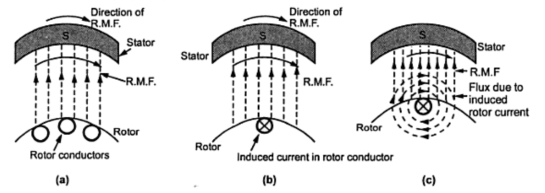### Working Principle of 3-Phase Induction Motor

Induction motor works on the principle of electromagnetic induction.
When a three phase supply is given to the three phase stator winding, a rotating magnetic field of constant magnitude is produced as discussed earlier. The speed of this rotation magnetic field is synchronous speed Ns r.p.m.

Where                      f = supply frequency.
p = Number of poles for which stator winding is wound.
This rotating field produces an effect of rotating poles around a rotor. Let direction of rotation of this rotating magnetic field is clockwise as shown in the Fig. 1(a).Fig. 1

Now at this instant rotor is stationary and stator flux R.M.F. is rotating. So its obvious that there exists a relative motion between the R.M.F. and rotor conductors. Now the R.M.F. gets cut by rotor conductors as R.M.F. sweeps over rotor conductors. Whenever conductors cuts the flux, e.m.f. gets induced in it. So e.m.f. gets induced in the rotor conductors called rotor induced e.m.f. This is electro-magnetic induction. As rotor forms closed circuit, induced e.m.f. circulates current through rotor called rotor current as shown in the Fig.1(b). Let direction of this current is going into the paper denoted by a cross as shown in the Fig. 1(b).
Any current carrying conductor produces its own flux. So rotor produces its flux called rotor flux. For assumed direction of rotor current, the direction of rotor flux is clockwise as shown in the Fig. 1(c). This direction can be easily determined using right hand thumb rule. Now there are two fluxes, one R.M.F. and other rotor flux. Both the fluxes interact with each as shown in the Fig. 1(d). On left of rotor conductor, two fluxes cancel each other to produce low flux area. As flux lines act as stretched rubber band, high flux density area exerts a push on rotor conductor towards low flux density area. So rotor conductor experience a force from left to right in this case, as shown in the Fig. 1(d), due to interaction of the two fluxes.
As all the rotor conductors experience a force, the overall rotor experiences a torque and starts rotating. So interaction of the two fluxes is very essential for a motoring action. As seen from the Fig. 1(d), the direction of force experienced is same as that of rotating magnetic field. Hence rotor starts rotating in the same direction as that of rotating magnetic field.Fig 1 (d

Alternatively this can be explained as : according to Lenz's law the direction of induced current in the rotor is so as to oppose the cause producing it. The cause of rotor current is the induced e.m.f. which is induced because of relative motion present between the rotating magnetic field and the rotor conductors. Hence to oppose the relative motion i.e. to reduce the relative speed, the rotor experiences a torque in the same direction as that of R.M.F. and tries to catch up the speed of the rotating magnetic field.
So,                Ns = Speed of rotating magnetic field in r.p.m.
N = Speed of rotor i.e. motor in r.p.m.
Ns - N = Relative speed between the two, rotating magnetic field and the rotor conductors.
Thus rotor always rotates in same direction as that of R.M.F.

Can N = Ns ?
When rotor starts rotating, it tries to catch the speed of rotating magnetic field.
If it catches the speed of the rotating magnetic field, the relative motion between rotor and the rotating magnetic field will vanish ( Ns - N = 0). In fact the relative motion is the main cause for the induced e.m.f. in the rotor. So induced e.m.f. will vanish and hence there can not be rotor current and the rotor flux which is essential to produce the torque on the rotor. Eventually motor will stop. But immediately there will exist a relative motion between rotor and rotating magnetic field and it will start. But due to inertia of rotor, this does not happen in practice and motor continues to rotate with a speed slightly less than the synchronous speed of the rotating magnetic field  in the steady state. The induction motor never rotates at synchronous speed. The  speed at which it rotates is hence called subsynchronous speed and motor sometimes called synchronous motor.
...                          N < Ns
So it can be said that rotor slips behind the rotating magnetic field produced by stator. The difference between the two is called slip speed of the motor.
Ns - N = Slip speed of the motor in r.p.m.
This speed decides the magnitude of the induction e.m.f. and the rotor current, which in turn decides the torque produced. The torque produced is as per the requirements of overcoming the friction and iron losses of the motor along with the torque demanded by the load on the rotor.

1.2.